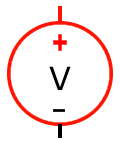# John Errington's tutorial on Power Supply Design

## Simple unregulated PSU - Worked ExampleThis example concludes the section on design for this unregulated supply (URS) by designing one to meet a particular specification.

The aim is to allow you to design your own URS which will become the first section of a voltage regulated supply.

### Specification

Design an unregulated supply that will be used to build a series voltage regulated bench supply with an output of up to 30V at 2A. The regulator circuit will have a 5V insertion loss. For reasons we will discuss later the ripple voltage will need to be kept to a sensible minimum.

Design considerations

OK so we need a MINIMUM voltage from the URS of 30V + 5V = 35V. Any additonal voltage above this will be dissipated as heat - which we dont want.

### Design process

Take the desired output = 35V and add 2V for losses in rectifier and trf = 37V
Now add the peak ripple. We will choose a 2V peak-peak ripple.

Vreq = 35 + 2 + 2 = 39V peak

#### Transformer

Calculate the RMS = 39 / 1.4 = 28V (30V is nearest sensible value)

so we need a 30V transformer at >2A. As this is for a high-spec unit we will allow a little extra in the VA rating. A quick look on the web shows 63VA or 100VA transformers are readily available - we will choose the 100VA.

A 30V transformer gives us 30*1.4 = 42V peak, and 40V after the rectifier. This only allows 40-35=5V peak to peak for ripple

#### Rectifier

Bridge rectifiers are inexpensive, so its no bad thing to choose one that exceeds the minimum requirements.

Current rating: take the I load and at least double it to allow for capacitor current. Here we need at least a 5A bridge.

Voltage rating: Do the same for the voltage here Vout=40V so pick V> 80V

An 8A 400V rectifier was only £0.48 and had a max forward voltage of "1.0V per element" = 2V.

### Smoothing

Here we do need to be cost-conscious as good high value caps with decent ripple current ratings are expensive.

Remember C = Iload / f * Vpk-pk ripple

so C = 2 / 100*5 = 4000 uF. We will choose a 4700uF capacitor rated at >40V

Remember the transformer voltage will rise if its load is removed -

if load regulation is 10% then Vmax peak = Vpk on-load * 1.1 = 42*1.1 = 47V

#### Ripple current

A search found a 10,000uF 80V capacitor rated at 8A ripple current.

This will give us a voltage ripple of Vpk-pk ripple= Iload / f C = 2/100*0.010 = 2V - well within our spec.